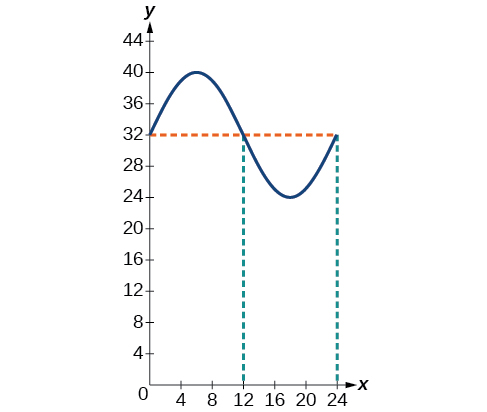# 7.6 Modeling with trigonometric equations  (Page 3/14)

 Page 3 / 14

## Describing periodic motion

The hour hand of the large clock on the wall in Union Station measures 24 inches in length. At noon, the tip of the hour hand is 30 inches from the ceiling. At 3 PM, the tip is 54 inches from the ceiling, and at 6 PM, 78 inches. At 9 PM, it is again 54 inches from the ceiling, and at midnight, the tip of the hour hand returns to its original position 30 inches from the ceiling. Let $\text{\hspace{0.17em}}y\text{\hspace{0.17em}}$ equal the distance from the tip of the hour hand to the ceiling $\text{\hspace{0.17em}}x\text{\hspace{0.17em}}$ hours after noon. Find the equation that models the motion of the clock and sketch the graph.

Begin by making a table of values as shown in [link] .

$x$ $y$ Points to plot
Noon 30 in $\left(0,30\right)$
3 PM 54 in $\left(3,54\right)$
6 PM 78 in $\left(6,78\right)$
9 PM 54 in $\left(9,54\right)$
Midnight 30 in $\left(12,30\right)$

To model an equation, we first need to find the amplitude.

The clock’s cycle repeats every 12 hours. Thus,

The vertical shift is

There is no horizontal shift, so $\text{\hspace{0.17em}}C=0.\text{\hspace{0.17em}}$ Since the function begins with the minimum value of $\text{\hspace{0.17em}}y\text{\hspace{0.17em}}$ when $\text{\hspace{0.17em}}x=0\text{\hspace{0.17em}}$ (as opposed to the maximum value), we will use the cosine function with the negative value for $\text{\hspace{0.17em}}A.\text{\hspace{0.17em}}$ In the form $\text{\hspace{0.17em}}y=A\text{\hspace{0.17em}}\mathrm{cos}\left(Bx±C\right)+D,\text{\hspace{0.17em}}$ the equation is

$y=-24\text{\hspace{0.17em}}\mathrm{cos}\left(\frac{\pi }{6}x\right)+54$

## Determining a model for tides

The height of the tide in a small beach town is measured along a seawall. Water levels oscillate between 7 feet at low tide and 15 feet at high tide. On a particular day, low tide occurred at 6 AM and high tide occurred at noon. Approximately every 12 hours, the cycle repeats. Find an equation to model the water levels.

As the water level varies from 7 ft to 15 ft, we can calculate the amplitude as

The cycle repeats every 12 hours; therefore, $\text{\hspace{0.17em}}B\text{\hspace{0.17em}}$ is

$\frac{2\pi }{12}=\frac{\pi }{6}$

There is a vertical translation of $\text{\hspace{0.17em}}\frac{\left(15+8\right)}{2}=11.5.\text{\hspace{0.17em}}$ Since the value of the function is at a maximum at $\text{\hspace{0.17em}}t=0,$ we will use the cosine function, with the positive value for $\text{\hspace{0.17em}}A.$

$y=4\text{\hspace{0.17em}}\mathrm{cos}\left(\frac{\pi }{6}\right)\text{\hspace{0.17em}}t+11$

The daily temperature in the month of March in a certain city varies from a low of $\text{\hspace{0.17em}}24\text{°F}\text{\hspace{0.17em}}$ to a high of $\text{\hspace{0.17em}}40\text{°F}\text{.}\text{\hspace{0.17em}}$ Find a sinusoidal function to model daily temperature and sketch the graph. Approximate the time when the temperature reaches the freezing point $\text{\hspace{0.17em}}32\text{°F}\text{.}\text{\hspace{0.17em}}$ Let $\text{\hspace{0.17em}}t=0\text{\hspace{0.17em}}$ correspond to noon.

$y=8\mathrm{sin}\left(\frac{\pi }{12}t\right)+32$
The temperature reaches freezing at noon and at midnight.## Interpreting the periodic behavior equation

The average person’s blood pressure is modeled by the function $\text{\hspace{0.17em}}f\left(t\right)=20\text{\hspace{0.17em}}\mathrm{sin}\left(160\pi t\right)+100,\text{\hspace{0.17em}}$ where $\text{\hspace{0.17em}}f\left(t\right)\text{\hspace{0.17em}}$ represents the blood pressure at time $\text{\hspace{0.17em}}t,$ measured in minutes. Interpret the function in terms of period and frequency. Sketch the graph and find the blood pressure reading.

The period is given by

In a blood pressure function, frequency represents the number of heart beats per minute. Frequency is the reciprocal of period and is given by

$\begin{array}{c}\frac{\omega }{2\pi }=\frac{160\pi }{2\pi }\\ =80\end{array}$

See the graph in [link] .

## Modeling harmonic motion functions

Harmonic motion is a form of periodic motion, but there are factors to consider that differentiate the two types. While general periodic motion applications cycle through their periods with no outside interference, harmonic motion requires a restoring force. Examples of harmonic motion include springs, gravitational force, and magnetic force.

a colony of bacteria is growing exponentially doubling in size every 100 minutes. how much minutes will it take for the colony of bacteria to triple in size
what is the importance knowing the graph of circular functions?
can get some help basic precalculus
What do you need help with?
Andrew
how to convert general to standard form with not perfect trinomial
can get some help inverse function
ismail
Rectangle coordinate
how to find for x
it depends on the equation
Robert
whats a domain
The domain of a function is the set of all input on which the function is defined. For example all real numbers are the Domain of any Polynomial function.
Spiro
foci (–7,–17) and (–7,17), the absolute value of the differenceof the distances of any point from the foci is 24.
difference between calculus and pre calculus?
give me an example of a problem so that I can practice answering
x³+y³+z³=42
Robert
dont forget the cube in each variable ;)
Robert
of she solves that, well ... then she has a lot of computational force under her command ....
Walter
what is a function?
I want to learn about the law of exponent
explain this
what is functions?
A mathematical relation such that every input has only one out.
Spiro
yes..it is a relationo of orders pairs of sets one or more input that leads to a exactly one output.
Mubita
Is a rule that assigns to each element X in a set A exactly one element, called F(x), in a set B.
RichieRich
If the plane intersects the cone (either above or below) horizontally, what figure will be created?

#### Get Jobilize Job Search Mobile App in your pocket Now!ByByBy Karen GowdeyBy Richley CrapoBy Sheila LopezByBy OpenStaxBy Vanessa SoledadBy OpenStaxByBy OpenStaxBy OpenStax